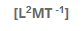# Angular Momentum

The measure of the quantity of motion possessed by a body in rotational motion is called angular momentum.

Definition
The angular momentum of a body about an axis is the product of its linear momentum and the perpendicular distance of the body from the axis.
In other words
The angular momentum of a body is equal to cross product of its
linear momentum and the vector distance from the axis of rotation.

Mathematical representation
If a body of mass “m” is moving in a circle or radius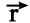with velocity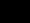the linear momentum of bodyis the angular momentum of the body is given by: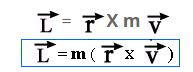Magnitude of Angular momentum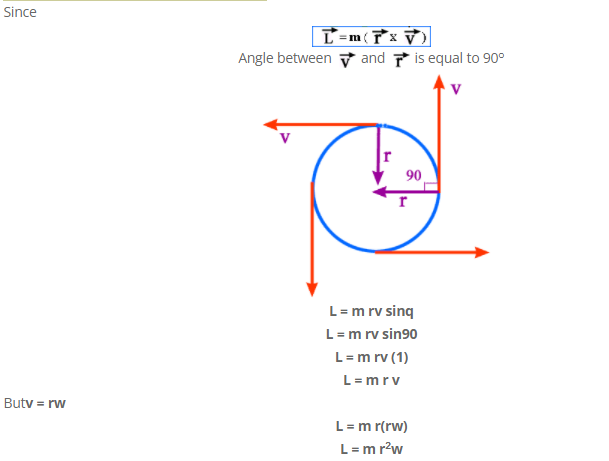We know that m r2 is equal to moment of inertia i.e. I = m r2
Thus,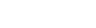Unit of angular momentum
In S.I. System unit of angular momentum is "joule.second".

Dimension of angular momentum